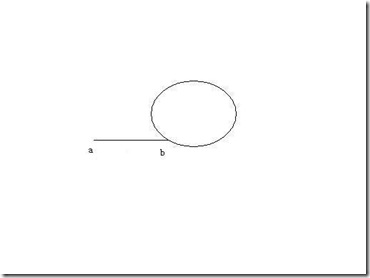# 归档 2010年10月

## 关于负载均衡和过载保护的一些想法和实现

1.轮询式

```vector vecServer;
while(1)
{
Server* server = vecServer[curIndex % vecServer.size()];
curIndex ++;
}
```

2.定死权重式

```//假设A，B, C server的权重分别为 10 5 2
typedef struct ...```

## python中对时间处理的几个函数

```time_t t = time(NULL);
```

```# -*- coding: utf-8 -*-

import time
import datetime

def StringToTime(strtime):
t_tuple = time.strptime(strtime,"%Y-%m-%d %H:%M:%S")
return time.mktime(t_tuple)

def StringToTime2(strtime):
dt = datetime.datetime.strptime(strtime,"%Y-%m-%d %H ...```

## 在C++中实现foreach循环，比for_each更简洁！

python,c#,java里面都有类似于foreach的结构，stl里面虽然有for_each这个函数，但是感觉使用还是太繁琐了一些，所以就自己实现了一个。 先来看看stl里面的for_each函数，官方文档上的原型如下：

``Function for_each (InputIterator first, InputIterator last, Function f);``

``````// for_each example
#include <iostream>
#include <algorithm>
#include <vector>
using namespace std;

void myfunction (int i) {
cout << " " << i;
}

struct myclass {
void operator() (int i) {cout << " " << i;}
} myobject;

int main () {
vector<int> myvector;
myvector.push_back(10);
myvector ...``````

## 一个简单的stl中string的split函数

``````int SplitString(const string &srcStr,const string &splitStr,vector<string> &destVec)
{
if(srcStr.size()==0)
{
return 0;
}
size_t oldPos,newPos;
oldPos=0;
newPos=0;
string tempData;
while(1)
{
newPos=srcStr.find(splitStr,oldPos);
if(newPos!=string::npos)
{
tempData = srcStr.substr(oldPos,newPos-oldPos);
destVec.push_back(tempData);
oldPos=newPos+splitStr ...``````

## 关于mysql_free_result和mysql_close的解惑

``````mysql_free_result(m_result);
mysql_close(m_Database);``````

1.当使用长连接时（即connect之后一直不close），如果最后会调用mysql_close，需不需要每次都调用mysql_free_result呢?
2.当mysql_close调用之后，m_result的数据是否还可以用。

2.还是可以使用。经过valgrind扫描，只调用mysql_close的扫描结果是：

``````==9397== 16,468 (88 direct, 16,380 indirect) bytes in 1 blocks are definitely lost in loss record 4 of 5
==9397==    at 0x40219B3: malloc (vg_replace_malloc.c:195)
==9397==    by ...``````

## 关于类成员函数指针的正确写法

``int innerFunc(int num);``

``````int (*ptrFunc)(int);
ptrFunc = innerFunc;//或&innerFunc``````

``````typedef int (*FUNC)(int);
FUNC ptrFunc;
ptrFunc = innerFunc;//或&innerFunc``````

``````#include <iostream>
#include <string>
#include <vector>
#include <map>
using namespace std;

class Foo
{
public:
string m_str;
Foo()
{
m_str = "";
}
void test(char* str,int num)
{
m_str = str ...``````

## 只能用Vim编程的笔记本## 一道不错的算法题-判断链表是否有环xt-yt=pn
yt>m

t=pn/(x-y) > m/y(只需pn可整除(x-y))